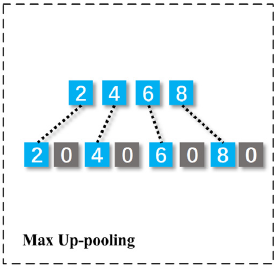# How to perform Max-up Pooling on tensor?

I am implementing SCAE. Which is a type of Convolutional Autoencoder

One of the operations in the decoder network is a Max Up-Pool layer (technically it is not a layer as it does not have wrights). Max Up Pool adds zeros at alternate positions in the upscaled tensor. This can be illustrated below:My input tensor’s shape is 1`* 4 * 64` and I need to upsample it to `1* 4 * 128` shape using the Max Up-pooing. I made the below function to perform max up pooling:

``````def maxUpPool(self, inpTensor):
up_sampled = torch.zeros(inpTensor.shape, inpTensor.shape, inpTensor.shape * 2)
up_sampled[:, :, ::2] = inpTensor
return up_sampled
``````

Is this the right approach to perform this operation ? I doubt if the back propagation will work since I have made a new tensor and it might not have the history of the operations performed on the `inpTensor`.

EDIT: Yes, the autograd engine is able to keep tracking history. So backpropagation is working. The approach suggested by @vainaijr is yielding similar results with the above mentioned approach.

maybe,

``````class CustomPool(nn.Module):
def __init__(self, size):
super().__init__()
self.size = size
def forward(self, x):
y = torch.repeat_interleave(x, self.size)
y.index_fill_(0, torch.arange(1, y.size(), self.size), 0)
print(y)
return y # or reshape it

model = nn.Sequential(CustomPool(2))
``````

but it would not work if we want more than one zero after each element

Tried this, but the model doesn’t seem to converge. It’s giving the same loss after every epoch.

``````class SCAE(nn.Module):

def __init__(self):
super().__init__()
# Encoding block:
self.conv1 = nn.Conv1d(in_channels = 1, out_channels = 2, kernel_size = 1)
self.conv2 = nn.Conv1d(in_channels = 2, out_channels = 16, kernel_size = 16)
self.conv3 = nn.Conv1d(in_channels = 16, out_channels = 4, kernel_size = 32)
self.conv4 = nn.Conv1d(in_channels = 4, out_channels = 8, kernel_size = 64)
self.conv5 = nn.Conv1d(in_channels = 8, out_channels = 4, kernel_size = 64)
self.maxpool = nn.MaxPool1d(kernel_size = 2)
self.conv6 = nn.Conv1d(in_channels = 4, out_channels = 2, kernel_size = 64)
self.batchnorm1d = nn.BatchNorm1d(num_features = 2)
self.conv7 = nn.Conv1d(in_channels = 2, out_channels = 1, kernel_size = 1)

# Decoding block:
self.convT1 = nn.ConvTranspose1d(in_channels = 1, out_channels = 2, kernel_size = 1)
self.convT2 = nn.ConvTranspose1d(in_channels = 2, out_channels = 4, kernel_size = 64)
self.convT3 = nn.ConvTranspose1d(in_channels = 4, out_channels = 8, kernel_size = 64)
self.convT4 = nn.ConvTranspose1d(in_channels = 8, out_channels = 4, kernel_size = 64)
self.convT5 = nn.ConvTranspose1d(in_channels = 4, out_channels = 2, kernel_size = 32)
self.convT6 = nn.ConvTranspose1d(in_channels = 2, out_channels = 2, kernel_size = 16)
self.convT7 = nn.ConvTranspose1d(in_channels = 2, out_channels = 1, kernel_size = 1)

def maxUpPool(self, inpTensor):
up_sampled = torch.repeat_interleave(inpTensor,2, dim = 2)
up_sampled.index_fill_(2, torch.arange(1, y.size(), 2), 0)
return up_sampled

def forward(self, x):

# Encoding:
enc = torch.tanh(self.conv1(x))
enc = torch.tanh(self.conv2(enc))
enc = torch.tanh(self.conv3(enc))
enc = torch.tanh(self.conv4(enc))
enc = torch.tanh(self.conv5(enc))
enc = self.maxpool(enc)
#         print(enc.shape)
enc = F.tanh(self.conv6(enc))
enc = F.tanh(self.conv7(enc))

# Decoding:
dec = torch.tanh(self.convT1(enc))
dec = torch.tanh(self.convT2(dec))
dec = self.maxUpPool(dec)
dec = torch.tanh(self.convT3(dec))
dec = torch.tanh(self.convT4(dec))
dec = torch.tanh(self.convT5(dec))
dec = torch.tanh(self.convT6(dec))
dec = torch.tanh(self.convT7(dec))
return dec.view(-1, 300)
``````

Is this the right way to use your suggested approach ?

EDIT: There was some problem in my training loop. Both the approaches are yielding similar results. The autograd engine is able to keep tracking history with slicing.